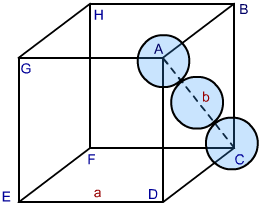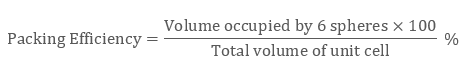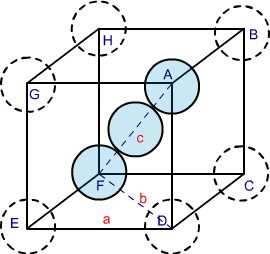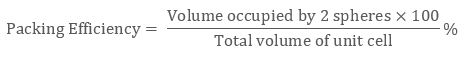# explain the packing efficiency of fcc,bcc,hcp?

` Dear Student, `

### HCP and CCP Structures

In both types of packing hexagonal closed packing or cubic closed packing the packing efficiency is same. In order to calculate packing efficiency in cubic closed structure, we consider a unit cell with edge length “a” and face diagonal AC to be “b”.Image 2: CCP Structure

Looking at the ABCD face of the cube, we see a triangle ABC. Let the radius of the each sphere ball be ‘r’. Correlating radius and edge of the cube, we proceed as follows:

In △ ABC, by Pythagoras theorem we can write:

AC2 = BC2 + AB2

Since AC = b and BC = AB = a, substituting the values in the above relation we get

b= a2 + a2

b2 = 2a2

b = √2 a….. (1)

also edge b in terms of radius ‘r’ equals

b = 4r  … (2)

From (1) and (2) we can write

4r = √2 a

or

a = 2 √2 r

As there are 4 spheres in a ccp structure unit cell, the total volume occupied by them will be

4 × 4 / 3 π r3

Also, total volume of a cube is (edge length)3 that is, ( a3) or in terms of r it is (2 √2 r)3, therefore packing efficiency will be:Therefore packing efficiency in fcc and hcp structures is 74%

Similarly in HCP lattice the relation between radius ‘r’ and edge length of unit cell “a” is r = 2a and number of atoms is 6.Body-Centred Cubic Structures

In body-centred cubic structures, the three atoms are diagonally arranged. To find the packing efficiency we consider a cube with edge length a, face diagonal length b and cube diagonal as c.Image 3: BCC structure

In △ EFD according to Pythagoras theorem

b= a2 + a2

b2 = 2a2

b  = √2 a

Now in △ AFD according to Pythagoras theorem

c= a2 + b2 = a2 + 2a2

c2 = 3a2

c  = √3 a

If the radius of each sphere is ‘r’ then we can write

c = 4r

√3 a = 4r

r = √3/ 4 a

As there are two atoms in the bcc structure the volume of constituent spheres will be

2 × (4/3) π r3Therefore packing efficiency of the body-centred unit cell is 68%.
Regards.

• 1
What are you looking for?